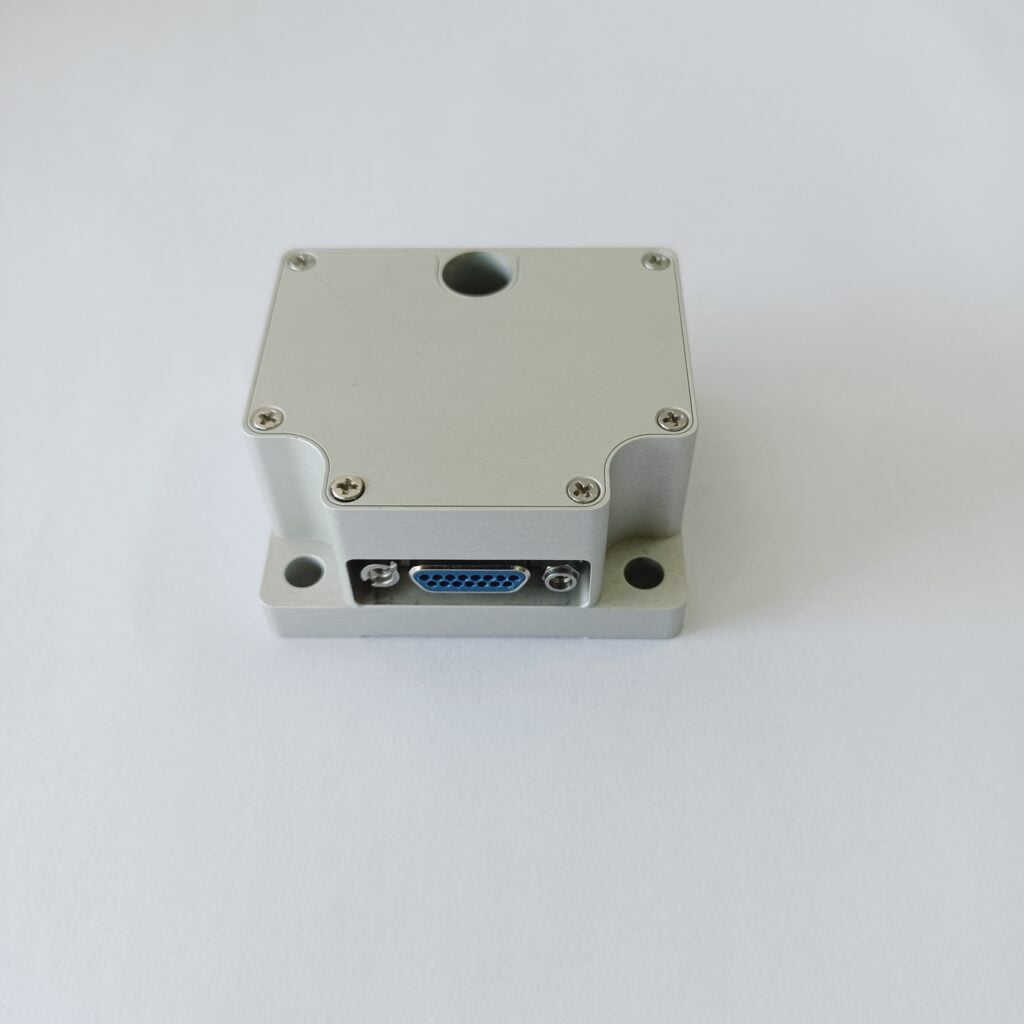Application

# What are the Components in IMU?IMU(Inertial Measurement Unit) is mostly used in devices that need motion control, such as cars and robots. It is also used in occasions that require precise displacement estimation with attitude, such as inertial navigation equipment of submarines, aircraft, missiles and spacecraft. The basic composition of IMU uses the three-axis geomagnetic solution combined with the three-axis accelerometer, which is greatly affected by the acceleration of external forces. In the environment of motion / vibration, the output direction angle error is large. This external magnetic sensor has shortcomings. Its absolute reference is the magnetic line of force of the geomagnetic field. Geomagnetism is characterized by a wide range of use, but low intensity, about a few tenths of a Gauss, which is very easy to be disturbed by other magnets. If the instantaneous angle of the z-axis gyroscope is integrated, It can make the system data more stable. The acceleration is measured in the direction of gravity. In the absence of external force acceleration, it can accurately output the roll/pitch two axis attitude angle, and this angle will not have cumulative error, which is accurate in a longer time scale

The whole inertial system is encapsulated by a metal shell, in which an IMU contains three single axis accelerometers and three single axis gyroscopes. The accelerometers detect the acceleration signals of the independent three axes of the object in the carrier coordinate system, and the gyroscopes detect the angular velocity signals of the carrier relative to the navigation coordinate system, measure the angular velocity and acceleration of the object in three-dimensional space, and then calculate the pose of the object.

In general, the components in IMU are: acceleration sensor, angular acceleration sensor, and some algorithm integrated circuits. Ericco has not only FOG IMU, but also MEMS IMU, high-precision navigation level and north seeking level. If you are interested, please feel free to contact us.